# Fisseha Berhane, PhD

#### Data Scientist443-970-2353[email protected] CV Resume## Anomaly Detection with R

Anomaly detection is used for different applications. It is a commonly used technique for fraud detection. It is also used in manufacturing to detect anomalous systems such as aircraft engines. It can also be used to identify anomalous medical devices and machines in a data center. You can read more about anomaly detection from Wikipedia.

In this blog post, I will implement anomaly detection algorithm and apply it to detect failing servers on a network. The data is from the famous Machine Learning Coursera Course by Andrew Ng. The lab exercises in that course are in Octave/Matlab. I am planning to do all the programming exercises in that course with R and I have started with anomaly detection.

First, we will start with 2D data and detect anomalous servers based on two features. We will plot the 2D data and see the algorithm's performance on a 2D plot. You can download the data for the first part of the exercise in RData format from here.

The features measure the through-put (mb/s) and latency (ms) of response of each server. We have 307 measurements.

We will use a gaussian (normal) model to detect anomalous examples in our dataset. We use multivariate normal distribution to detect servers with very low probabilities and hence can be considered anomalous (outliers). You can read about multivariate normal distribution from Wikipedia.

The probability density function (PDF) of a multivariate normal is $$f(x)=(2\pi)^\frac{-k}{2} |\Sigma|^\frac{-1}{2}e^{-1/2(x-\mu)^{'} \Sigma^{-1}(x-\mu)}$$

$\Sigma$ is variance and $|\Sigma|$ is determinant of the variance. $\mu$ is mean and $k$ is number of columns (variables) of our data.

In :
library(magrittr)  # to use piping %>%
library(ggplot2)   # for ploting
library(MASS)      # to calculate the pseudo-inverse of a matrix
library(caret)     # to center our data by subtracting its mean
library(reshape2)  # for data manipulation

In :
load("data1.RData")

In :
#All the variables below are matrices

X=data1$X Xval=data1$Xval   # This is cross-validation data
yval =data1$yval # This shows which rows in Xval are anomalous  In : head(X)   13.0468 14.7411 13.4085 13.7633 14.1959 15.8532 14.9147 16.1743 13.5767 14.0428 13.9224 13.4065 In : head(Xval)   15.7903 14.921 13.6396 15.33 14.8659 16.4739 13.5847 13.9893 13.464 15.6353 12.9489 16.1401 In : table(yval)  yval 0 1 298 9  From the cross-validation data, we have only nine anomalous servers but there are 298 normal servers. The main reason we can not use supervised machine learning techniques in such scenarious is because we have only a small proportion of the data as anomalous We will use a gaussian model to detect anomalous examples in our dataset. So, first, let's check the distribution of the variables and see if they are approximately normally distributed. If they are not close to normal distribution, we have to use appropriate transformations to get good approximations of normal distribuions. In : X=as.data.frame(X) names(X)=c("Latency (ms)", "Throughput (mb/s)") XX=melt(X)  In : XX%>%ggplot(aes(x=value,fill=variable, color=variable))+ geom_density(alpha = 0.3)+ggtitle('Distibution of X')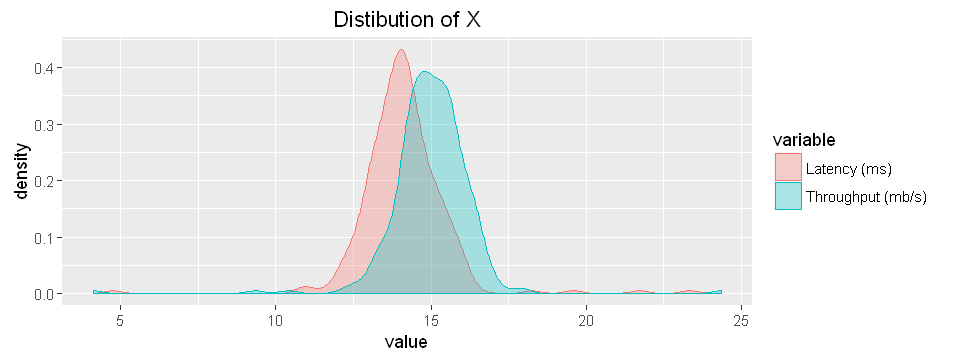As we can see, normal distribution is a good approximation for our dataset and hence we do not need to perform any transformations. Next, let's see the distribution of the cross-validation data (Xval). In : Xval=as.data.frame(Xval) names(Xval)=c("Latency (ms)", "Throughput (mb/s)") XXval = melt(Xval) XXval%>%ggplot(aes(x=value,fill=variable, color=variable))+ geom_density(alpha = 0.3)+ggtitle('Distibution of Xval')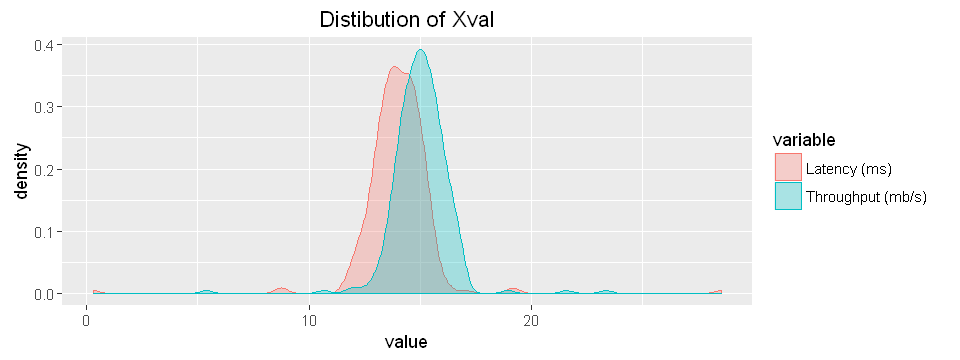The variables in Xval are also close to gaussian distribution. So, we do not need any transformation. Now, let's create a 2D plot and see the distribution of Latency and Throughput. In : X%>%ggplot(aes(x=Latency (ms),y=Throughput (mb/s)))+ geom_point(color='blue')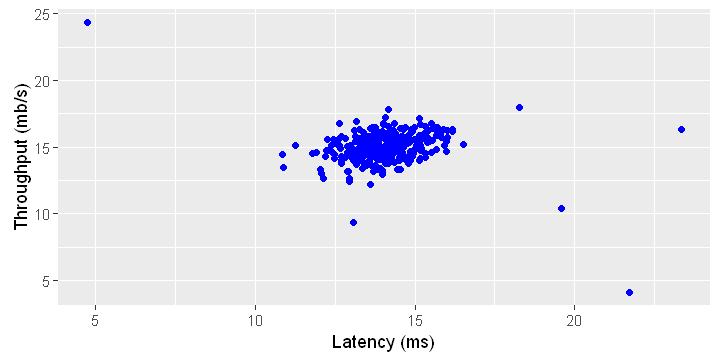From the 2D plot above, we can visually detect the outliers and let's see if our algirithm can detect them. Remeber, the main advantage of anomaly detection algortims is specially when we have more than two measured features which is the case most of the time. Again, the probability density finction (PDF) is given by $$PDF=(2\pi)^\frac{-k}{2} |\Sigma|^\frac{-1}{2}e^{-1/2(x-\mu)^{'} \Sigma^{-1}(x-\mu)}$$$\Sigma $is variance and$|\Sigma|$is determinant of the variance. In the PDF above, we have$(x-\mu)$, so, let's use the caret package to subtract the mean from the data points. This is called centering. In : # Create preProcess object preObj <- preProcess(X,method="center") # Center the data- subtract the column means from the data points X2 <- predict(preObj,X)  Now,let's calculate variance. We will make it a diagonal matrix. In : X2= as.matrix(X2) sigma2=diag(var(X2)) sigma2  Latency (ms) 1.83862040504188 Throughput (mb/s) 1.7153327338707 In : sigma2=diag(sigma2) sigma2   1.83862 0 0 1.71533 Now, let's calculate the probabilities using the multivariate normal distribution equation given above. In : A=(2*pi)^(-ncol(X2)/2)*det(sigma2)^(-0.5) B = exp(-0.5 *rowSums((X2%*%ginv(sigma2))*X2)) p=A*B  #### Now, let's explore the probabilities¶ In : p= p%>%as.data.frame() names(p)= c('probability') p%>%ggplot(aes(probability))+geom_density(fill='skyblue')+ ggtitle('Distibution of calculated probabilities')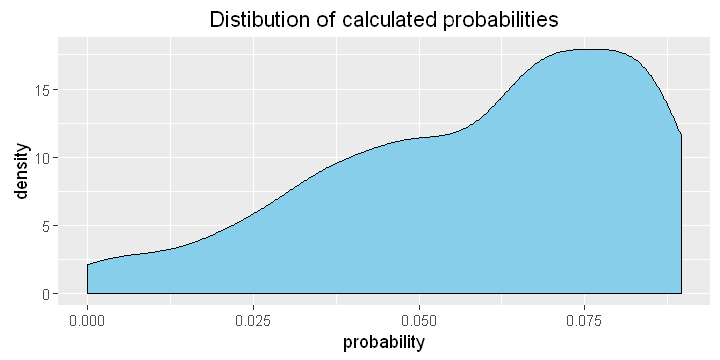From the density plot above, we see there are some computer servers with very low probabilities which could be outliers. We can also plot a box plot of the probabilities. Here, I am also showing the points and this helps us to see the probabilities of each server. In : p%>%ggplot(aes(y=probability,x=1))+geom_boxplot()+ geom_jitter()+xlab('')+ggtitle("Box plot of calculate probabilities")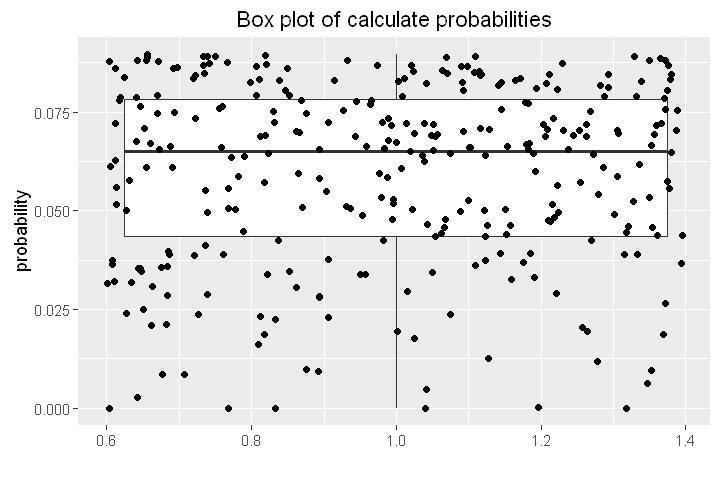We can also create a contour plot of the probabilities and see where the servers with highest probabilities are located. In : X= cbind(X,p) ggplot(X, aes(x=Latency (ms), y=Throughput (mb/s), z=probability))+ geom_point()+ stat_density2d(color='red')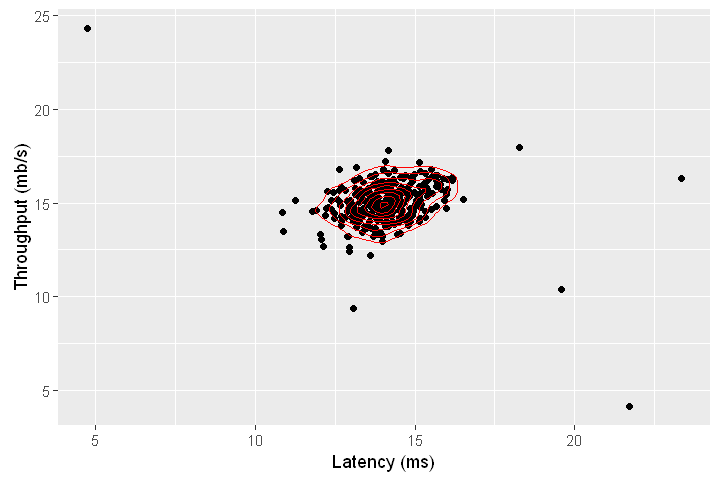From the contour plot shown above, we see that most points are near the center and the contours show relatively higher probabilities in this region. We can see that the outliers are dispersed far away from the center of mass. When we are working with 2D data, we may be able to identify the outliers visually but this techniques is specially very useful when we are working with data with more than two dimensions. To determine which of the points shown in the figure above are anomalous, we have to use cross-validation. From cross-validation, we can get the cut-off probability to classify the servers as normal or anomalous. In data1.RData, Xval is the validation data and yval is the actual observation of the validation data (whether the servers were anomalous or not). We will use F1 score measure to get the threshould probability and also the accuracy of our model. First, let's remove mean of each column from Xval. In : # Create preProcess object Xval = as.data.frame(Xval) preObj <- preProcess(Xval,method="center") # Center the data- subtract the column means from the data points Xval_centered <- predict(preObj,Xval)  Then, calculate its variance. In : Xval_centered = as.matrix(Xval_centered) sigma2=diag(var(Xval_centered)) sigma2 = diag(sigma2) sigma2   2.5261 0 0 1.63133 Now, let's calculate pval of the cross-validation data Xval. In : A=(2*pi)^(-ncol(Xval_centered)/2)*det(sigma2)^(-0.5) B = exp(-0.5 *rowSums((Xval_centered%*%ginv(sigma2))*Xval_centered)) pval = A*B  For cross-validation, let's use F1 score, which uses precision and recall. Precision and rcall in turn are calculated using true positive, false positive and false negative as defined below. tp is the number of true positives: the ground truth label says it's an anomaly and our algorithm correctly classied it as an anomaly.  fp is the number of false positives: the ground truth label says it's not an anomaly, but our algorithm incorrectly classied it as an anomaly.  fn is the number of false negatives: the ground truth label says it's an anomaly, but our algorithm incorrectly classied it as not being anoma- lous. The F1 score is computed using precision (prec) and recall (rec): $$prec = \frac{tp}{tp+fp}$$ $$rec = \frac{tp}{tp+fn}$$ $$F1 = 2.\frac{prec.rec}{prec+rec}$$ The code chunk below calculates the probability cutt-off value for the maximum possible F1 score through cross-validation. In : bestEpsilon = 0 bestF1 = 0 F1 = 0 stepsize = (max(pval) - min(pval)) / 1000 for (epsilon in seq(from =min(pval), by= stepsize,to =max(pval))){ predictions = (pval < epsilon)*1 tp = sum((predictions == 1) & (yval == 1)) fp = sum((predictions == 1) & (yval == 0)) fn = sum((predictions == 0) & (yval == 1)) prec = tp / (tp + fp) rec = tp / (tp + fn) F1 = (2 * prec * rec) / (prec + rec) if (!is.na(F1)& (F1 > bestF1)==TRUE){ bestF1 = F1 bestEpsilon = epsilon } } cat("\n bestF1 =",round(bestF1,4)) cat("\n bestEpsilon =",round(bestEpsilon,4))   bestF1 = 0.875 bestEpsilon = 2e-04 So, from the result above, the best F1 score from the cross-validation is 0.875. We can use the bestEpsilon above as cut-off to classify the servers as anomalous or normal. In : X$outliers= X$probability < bestEpsilon X$outliers=as.factor(X$outliers) head(X)  Latency (ms)Throughput (mb/s)probabilityoutliers 113.0468151687048 14.7411524132184 0.0645666528169585FALSE 213.4085201853932 13.7632696002405 0.0502352510157861FALSE 314.195914812454915.85318112982810.0722651610036 FALSE 414.914700765313 16.1742598671581 0.0502467728286949FALSE 513.5766996051752 14.0428494375565 0.0635488729469846FALSE 613.9224025075003 13.4064689366608 0.0424235784705763FALSE Let's see how many outliers we have according to our model. In : table(X$outliers)

FALSE  TRUE
301     6 

We see that we have six outliers and 301 normal servers.

Let's visualize which servers are outliers.

In :
X%>%ggplot(aes(x=Latency (ms),y=Throughput (mb/s)))+
geom_point(aes(color=outliers))+ggtitle('Anomaly Detection')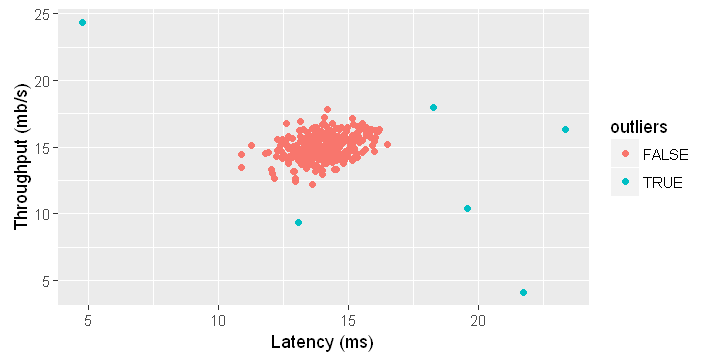As we can see from the figure, the results of our model make sense and now we can use it for data with any number of features.

### Multidimensional Outliers¶

We will now use the code from the previous part on a more realistic and much harder dataset. In this dataset, each example is described by 11 features, capturing many more properties of our compute servers. You can download the RData from here.

In :
load("data2.RData")

In :
X=data2$X # has 11 columns Xval=data2$Xval   # This is cross-validation data
yval =data2$yval # This shows which rows in Xval are anomalous  First, let's explore the distribution of the variables. In : X%>%as.data.frame()%>%melt()%>% ggplot(aes(x=value,color=variable))+geom_density()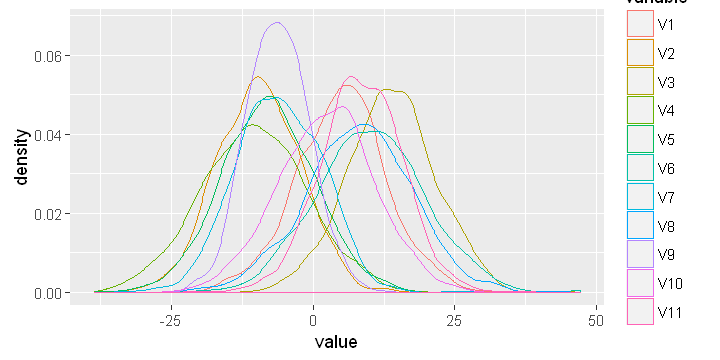Normal distribution seems a good approximation for this data. So, we do not need to perform any transformations. In : cat("Number of variables and observations of X") dim(X)  Number of variables and observations of X 1. 1000 2. 11 In : cat("Number of variables and observations of cross-validation data") dim(Xval)  Number of variables and observations of cross-validation data 1. 100 2. 11 In : cat("Labels of cross-validation data") cat("\ny=0 corresponds to normal servers and y=1 corresponds to anomalous servers") dim(yval)  Labels of cross-validation data y=0 corresponds to normal servers and y=1 corresponds to anomalous servers 1. 100 2. 1 The Xval measures are also nearly normally distributed as shown below. In : Xval%>%as.data.frame()%>%melt()%>% ggplot(aes(x=value,color=variable))+geom_density()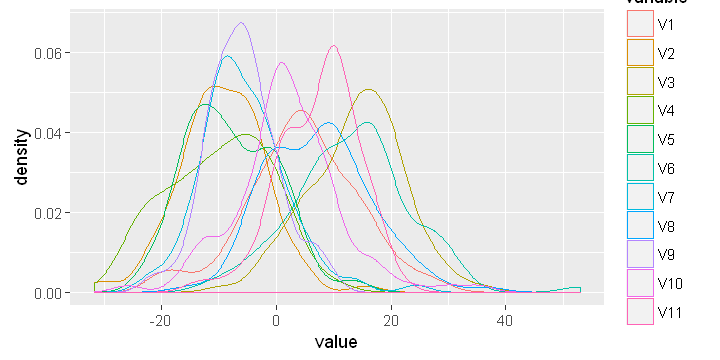Now, following the steps we used above, let's calculate probabilities for X and Xval. In : # Create preProcess object X = as.data.frame(X) preObj <- preProcess(X,method="center") # Center the data- subtract the column means from the data points X2 <- predict(preObj,X)  In : sigma2=cov(X2) sigma2=diag(sigma2) sigma2=diag(sigma2)  Calculate probability for X: In : X2= as.matrix(X2) A=(2*pi)^(-ncol(X2)/2)*det(sigma2)^(-0.5) B = exp(-0.5 *rowSums((X2%*%ginv(sigma2))*X2)) p = A*B  Next, let's calculate probabilties for the cross-validation data (Xval). In : # Create preProcess object Xval = as.data.frame(Xval) preObj <- preProcess(Xval,method="center") # Center the data- subtract the column means from the data points Xval_centered <- predict(preObj,Xval)  In : Xval_centered = as.matrix(Xval_centered) sigma2=diag(var(Xval_centered)) sigma2= diag(sigma2)  In : A=(2*pi)^(-ncol(Xval_centered)/2)*det(sigma2)^(-0.5) B = exp(-0.5 *rowSums((Xval_centered%*%ginv(sigma2))*Xval_centered)) pval = A*B  Now, we can determine the best probability threshold and also the associated F1 score. In : bestEpsilon = 0 bestF1 = 0 F1 = 0 stepsize = (max(pval) - min(pval)) / 1000 for (epsilon in seq(from =min(pval), by= stepsize,to =max(pval))){ predictions = (pval < epsilon)*1 tp = sum((predictions == 1) & (yval == 1)) fp = sum((predictions == 1) & (yval == 0)) fn = sum((predictions == 0) & (yval == 1)) prec = tp / (tp + fp) rec = tp / (tp + fn) F1 = (2 * prec * rec) / (prec + rec) if (!is.na(F1)& (F1 > bestF1)==TRUE){ bestF1 = F1 bestEpsilon = epsilon } } cat("\n bestF1 =",bestF1) cat("\n bestEpsilon =",bestEpsilon)   bestF1 = 0.64 bestEpsilon = 1.597136e-18 Finally, let's calculate the number of anomalous and normal servers.' In : probability=p X=cbind(X,probability) X$outliers= X$probability < bestEpsilon X$outliers=as.factor(X$outliers)  In : table(X$outliers)

FALSE  TRUE
869   131 

#### Summary¶

Anomaly detection has various applications ranging from fraud detection to anomalous aircraft engine and medical device detection. In this blog post, we used anomaly detection algorithm to detect outliers of servers in a network using multivariate normal model. This blog post in an R version of a machine Learning programming assignment with Matlab on Coursera offered by Andrew Ng.